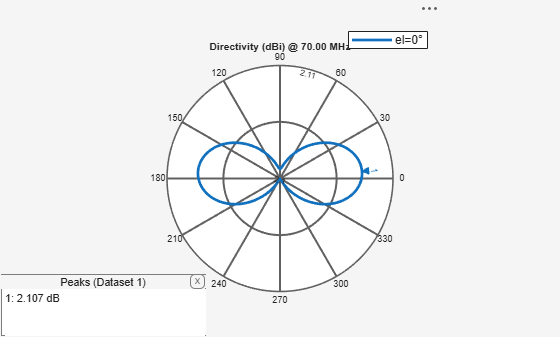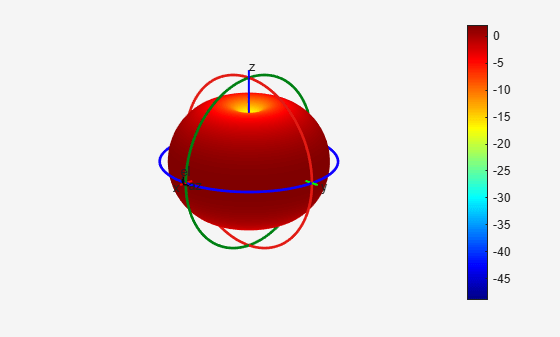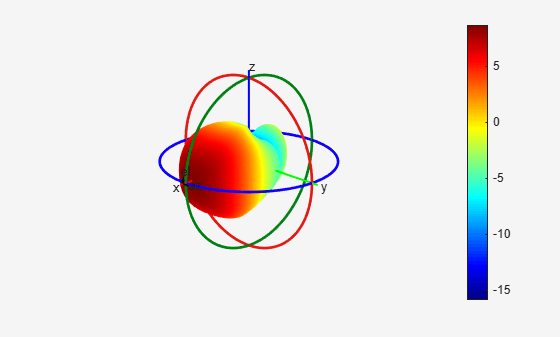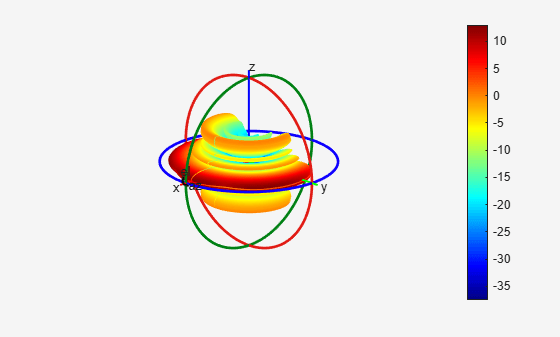Documentation

## 3D Reconstruction of Radiation Pattern From 2D Orthogonal Slices

This example shows how to reconstruct 3D radiation pattern using patternFromSlices function. A 3D radiation pattern is very important tool for antenna analysis, characterization, design, planning, and applications. This example will show reconstruction of 3-D radiation from 2 orthogonal slices. Pattern reconstruction for an omni-directional and directional antenna will be considered.

### Omni-Directional Antenna

Define an omni-directional antenna such as a dipole with a specific frequency and required elevation and azimuth angle.

```ant = dipole; freq = 70e6; ele = -90:5:90; azi = -180:1:180; ```

### Generate Orthogonal 2-D Slices.

The slice is along the vertical direction using patternElevation function. Here we can give other 2-D pattern data also.

```vertSlice = patternElevation(ant,freq,0,'Elevation',ele); theta = 90 - ele; ```

The two orthogonal slices can also be visualized.

```figure; patternElevation(ant,freq,0,'Elevation',ele); figure; patternAzimuth(ant,freq,0,'Azimuth',azi); ```### Reconstruction Of 3-D Radiation Pattern

For an omni-directional antenna, we can reconstruct the 3-D pattern using vertSlice alone. When only the elevation pattern data is provided, function assumes omnidirectionality of the antenna with symmetry about the z-axis (i.e., azimuthal symmetry).

```patternFromSlices(vertSlice,theta); ```Reconstruction using both vertSlice & horizSlice data points can also be done for the above case. The reconstructed pattern will not vary. Thus, for any omni-directional antenna 3-D pattern can be reconstructed with enough data points from orthogonal slices along the theta direction. The reconstructed radiation pattern looks like the 3-D radiation pattern which can be obtained using the pattern function.

### Discarding of Data Points

Discarding of data points during reconstruction of 3-D pattern happens when both data points span across 360 degrees in 2-D plane. Since the algorithm need maximum span of 360 degree in one plane and a span of 180 degree in the other plane, extra data points are discarded.

```vertSlice = patternElevation(ant,freq); theta = 90 - (-180:1:180); ```

Dimension of pat3-D won't be equal to the length(phi)*length(theta) in this case. The size of thetaout also vary from that of theta dimension. Also, thetaout data will show the values for which data points have been considered during reconstruction.

```[pat3D,thetaout]=patternFromSlices(vertSlice,theta); dim_theta = size(thetaout); disp(dim_theta); ```
``` 1 181 ```

3-D radiation pattern will not get affected by data discarding since there will be enough data points along both the orthogonal plane for reconstruction of 3-D pattern. This result will be the same as that of the above reconstructed 3-D radiation pattern.

```patternFromSlices(vertSlice,theta); ```### Directional Antenna

Define a directional antenna such as helix with specific frequency and values for elevation and azimuth angles.

```ant_dir = helix('Tilt',90,'TiltAxis',[0 1 0]); freq = 2e9; ele = -90:5:90; azi = -180:5:180; ```

### Orthogonal 2-D Slices

The slice along the vertical direction using patternElevation function.

```vertSlice = patternElevation(ant_dir,freq,0,'Elevation',ele); theta = 90 - ele; ```

The slice along the horizontal direction using patternAzimuth function.

```horizSlice = patternAzimuth(ant_dir,freq,0,'Azimuth',azi); phi = azi ; ```

The two orthogonal slices can also be visualized.

```figure; patternElevation(ant_dir,freq,0,'Elevation',ele); figure; patternAzimuth(ant_dir,freq,0,'Azimuth',azi); ```### Reconstruction Of 3-D Radiation Pattern

For a directional antenna pattern, both the horizontal and vertical slice must be provided for accurate pattern reconstruction. Two separate algorithms are implemented for pattern reconstruction and will consider both below.

### Summing Method.

The "classic" summing algorithm is the default method. This algorithm can be used for near-perfect reconstruction of omni-directional antennas than for directional antenna.

```patternFromSlices(vertSlice,theta,horizSlice,phi); ```### CrossWeighted Method.

In this algorithm, the normalization parameter can be changed to obtain different results for the reconstructed pattern about the estimated directivity/gain

```patternFromSlices(vertSlice,theta,horizSlice,phi,'Method','CrossWeighted'); ```### 3-D Radiation Using Pattern Function

Originally 3-D radiation pattern using pattern function for helix

```figure; pattern(ant_dir,freq); ```From comparing the above 3-D radiation pattern using pattern function and the reconstructed 3-D pattern it is clear that front plane of the 3-D pattern is reconstructed well compared to the back plane of it. Also, when reconstruction done using CrossWeighted method is more accurate then summing method for this case.

### Read and Visualize Antenna Data from Manufacturer

Antenna manufacturers typically provide details of the antennas that they supply together with the two orthogonal slices of the radiation pattern. The pattern data is available in a variety of formats. One such format that is supported in the Antenna Toolbox is the MSI file format (extension .msi or .pln). Use the msiread function to read the data into the workspace.

```[Horizontal,Vertical,Optional] = msiread('Test_file_demo.pln'); ```

### Adjust To dBi if data is in dBd

```if strcmpi(Optional.gain.unit,'dBd') Horizontal.Magnitude = Horizontal.Magnitude + 2; Vertical.Magnitude = Vertical.Magnitude + 2; end ```

Visualize the vertical and horizontal gain data in an interactive 2-D polar plot.

```figure P = polarpattern(Vertical.Elevation, Vertical.Magnitude); P.TitleTop = 'MSI Planet file data'; createLabels(P,'az=0#deg'); figure Pel = polarpattern(Horizontal.Azimuth, Horizontal.Magnitude); Pel.TitleTop = 'MSI Planet file data'; createLabels(Pel,'el=0#deg'); ```### Reconstruction Of 3-D Radiation Pattern

Extract the pattern slice magnitude data from the two output structures as well as the azimuth and elevation angle data. Note, that the angle data should be adjusted for the phi-theta convention. The azimuth angles maps to phi but the elevation angle is adjusted by 90 degrees to map to theta.

```vertSlice = Vertical.Magnitude; theta = 90-Vertical.Elevation; horizSlice = Horizontal.Magnitude; phi = Horizontal.Azimuth; patternFromSlices(vertSlice,theta,horizSlice,phi,'Method','CrossWeighted'); ```